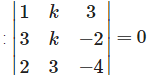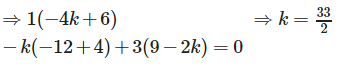# Test: Matrices (CBSE Level) - 2

## 25 Questions MCQ Test Mathematics (Maths) Class 12 | Test: Matrices (CBSE Level) - 2

Description
This mock test of Test: Matrices (CBSE Level) - 2 for JEE helps you for every JEE entrance exam. This contains 25 Multiple Choice Questions for JEE Test: Matrices (CBSE Level) - 2 (mcq) to study with solutions a complete question bank. The solved questions answers in this Test: Matrices (CBSE Level) - 2 quiz give you a good mix of easy questions and tough questions. JEE students definitely take this Test: Matrices (CBSE Level) - 2 exercise for a better result in the exam. You can find other Test: Matrices (CBSE Level) - 2 extra questions, long questions & short questions for JEE on EduRev as well by searching above.
QUESTION: 1

###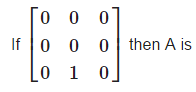Solution:

A square matrix A for which An= 0 , where n is a positive integer, is called a Nilpotent matrix.

QUESTION: 2

###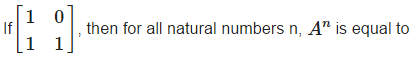Solution: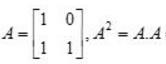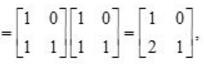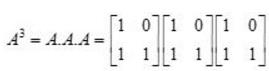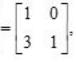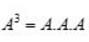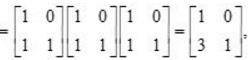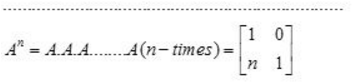QUESTION: 3

### If A any square matrix then which of the following is not symmetric ?

Solution:

For every square matrix (A – A’) is always skew – symmetric.

QUESTION: 4

Let a, b, c, d, u, v be integers. If the system of equations, a x + b y = u, c x + dy = v, has a unique solution in integers, then

Solution: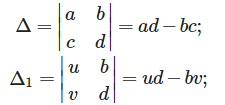ax + by = u , cx +dy = v ,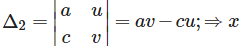since the solution is unique in integers.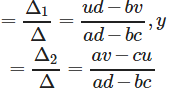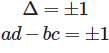QUESTION: 5

The system of equations, x + y + z = 1, 3 x + 6 y + z = 8, αx + 2 y + 3z = 1 has a unique solution for

Solution:

The given system of equations has unique solution , if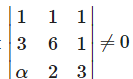⇒1(18−2)−1(9−α) ⇒13−5α ≠ 0 ⇒ α ≠ 13/5 + 1(6−6α) ≠ 0
Therefore , unique solution exists for all integral values of α.

QUESTION: 6

If A and B are symmetric matrices of the same order, then

Solution:

If A and B are symmetric matrices of the same order, then , AB + BA is always a symmetric matrix.

QUESTION: 7

If A = [aij]2×2 where aij= i + j, then A is equal to

Solution:

If A = [aij]2x2 where aij = i + j, then,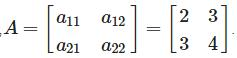QUESTION: 8

Each diagonal element of a skew-symmetric matrix is

Solution:

The diagonal elements of a skew-symmetric is zero.

QUESTION: 9

The system of equations, x + y + z = 6, x + 2 y + 3 z = 14, x + 3 y + 5z = 20 has

Solution:

The given system of equations does not has a solution if :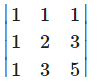0 ⇒ 1(10 -9) - 1(5-3) + 1(3-2)

= 0 ⇒ 1-2 + 1 = 0

QUESTION: 10

The matrix of the transformation ‘reflection in the line x + y = 0 ‘ is

Solution:

Let x' and y' be the reflection of x and y, therefore :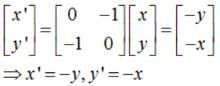Hence, reflection is on the line - x-y = 0⇒ x + y = 0

QUESTION: 11

A square matrix A = [aij]n×n is called a diagonal matrix if aij = 0 for

Solution:

In a diagonal matrix all elements except diagonal elements are zero.i.e.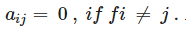QUESTION: 12

If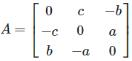and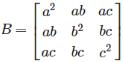then AB =

Solution: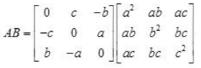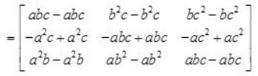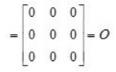QUESTION: 13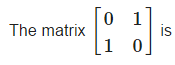Solution:

or a symmetric matrix A’ = A . therefore ,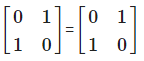QUESTION: 14

If In is the identity matrix of order n, the (In)−1

Solution:

Inverse of any identity matrix is always an identity matrix.

QUESTION: 15

If A = [x y z],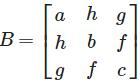and C = [xyt]t, then ABC is

Solution: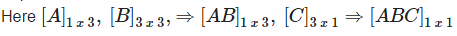QUESTION: 16

If a square matrix A has two identical rows or columns , then det.A is :

Solution:

Det.A = 0.

QUESTION: 17

For a skew symmetric odd ordered matrix A of integers, which of the following will hold true:

Solution:

Determinant of a skew symmetric odd ordered matrix A is always 0 .

QUESTION: 18

Matrix A when multiplied with Matrix C gives the Identity matrix I, what is C?

Solution:

Any square matrix when multiplied with its inverse gives the identity matrix. Note that non square matrices are not invertible.

QUESTION: 19

Let for any matrix M ,M−1exist. Which of the following is not true.

Solution:

Clearly , (M−1)−1 = (M−1)1 is not true.

QUESTION: 20

Rank of a non-zero matrix is always

Solution:

Rank of a non zero matrix is always greater than or equal to 1.

QUESTION: 21

For a non-trivial solution | A | is

Solution:

An n×n homogeneous system of linear equations has a unique solution (the trivial solution) if and only if its determinant is non-zero. If this determinant is zero, then the system has an infinite number of solutions. i.e. For a non-trivial solution ∣A∣=0.

QUESTION: 22

If for a matrix A, A2+I = O where I is the identity matrix, then A equals

Solution: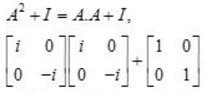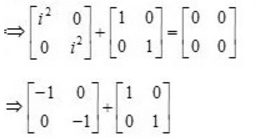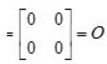QUESTION: 23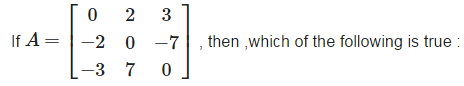Solution:

The given matrix is a skew – symmetric matrix.,therefore , A = - A’.

QUESTION: 24

The system of linear equations x + y + z = 2, 2x + y - z = 3, 3x + 2y - kz = 4 has a unique solution if ,

Solution:

The given system of equation has a unique solution if :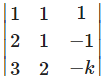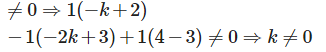QUESTION: 25

The value of k for which the system of equations, x + k y + 3 z = 0, 3 x + k y – 2 z = 0, 2 x + 3 y – 4 z = 0, have a non-trival solution is

Solution:

The given system of equations has a non-trivial solution if  :